Anzeige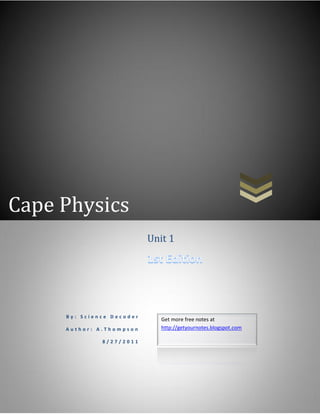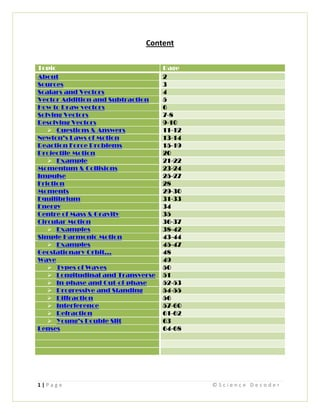Anzeige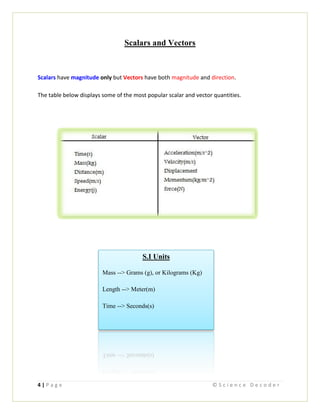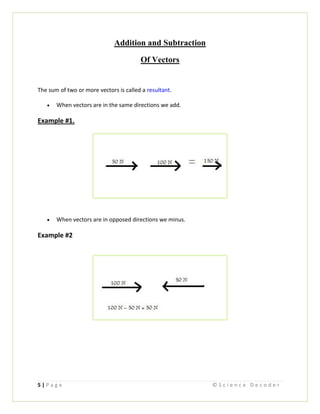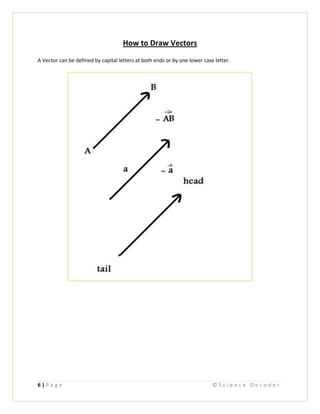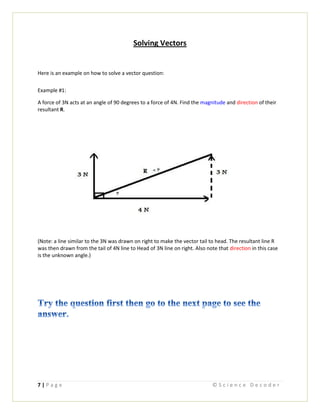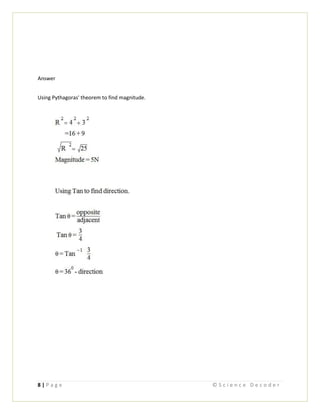Anzeige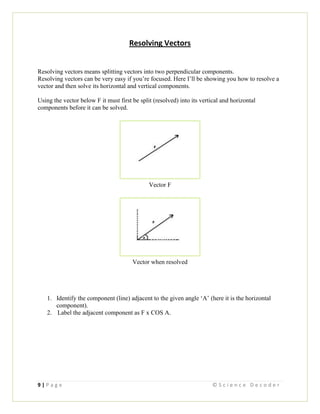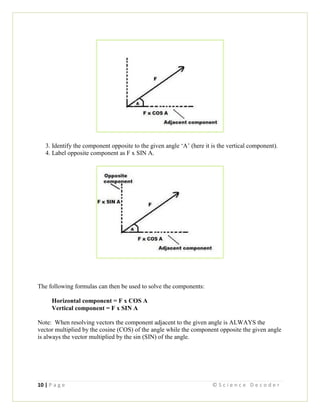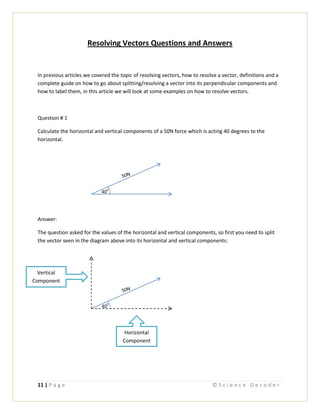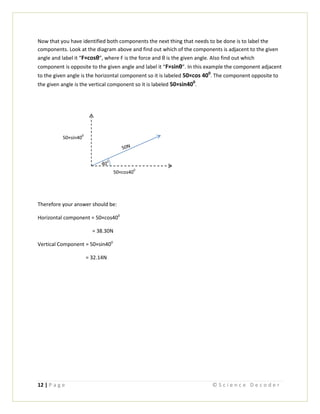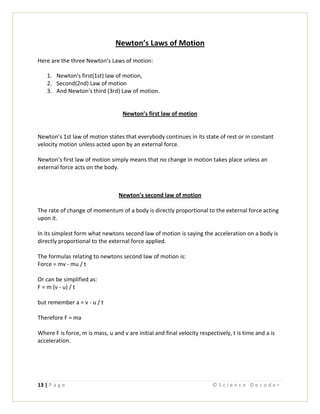Anzeige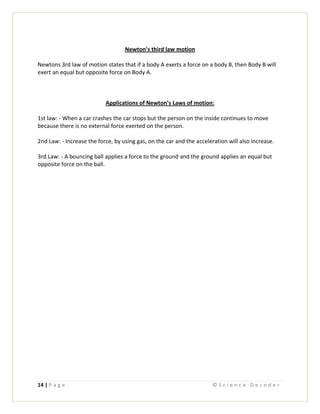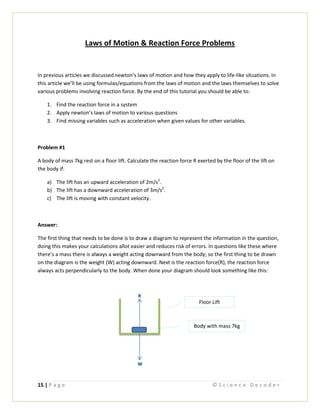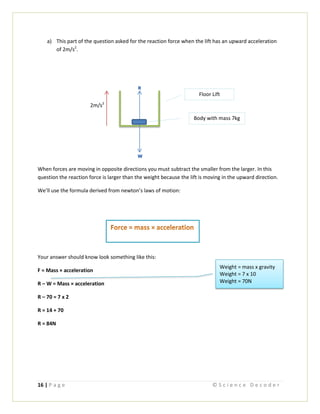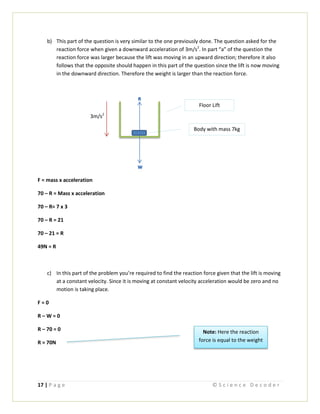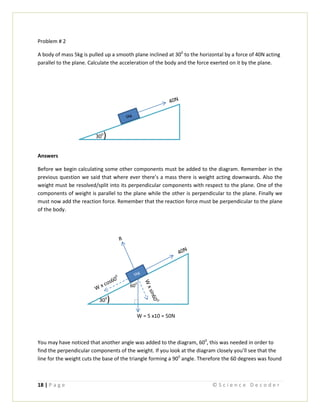Anzeige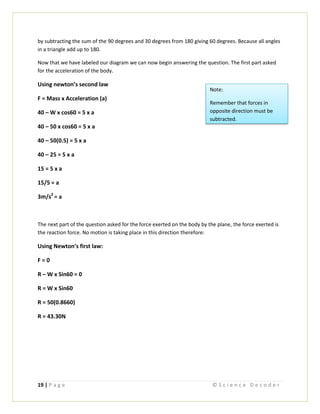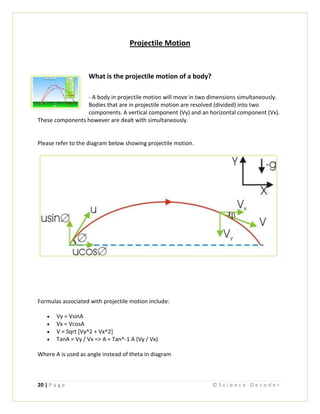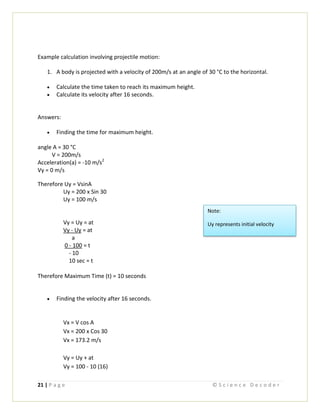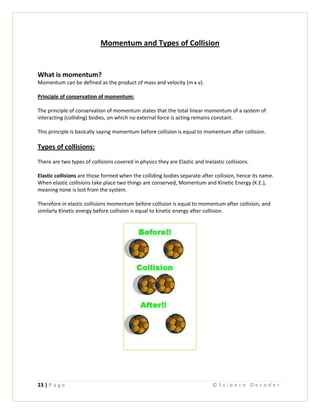Anzeige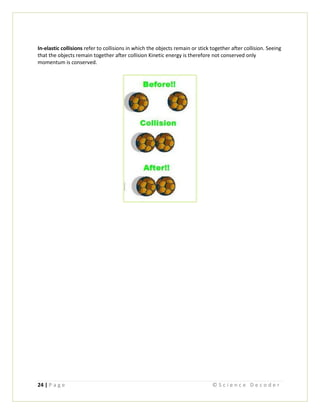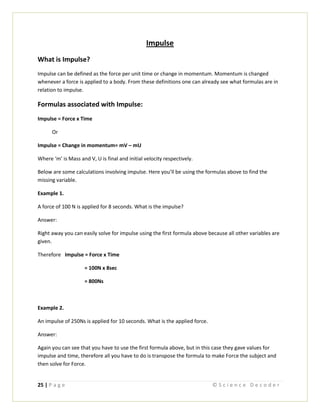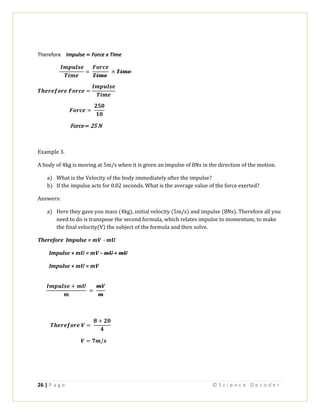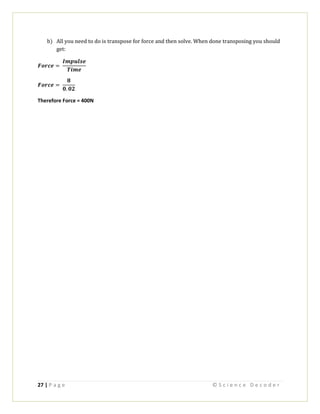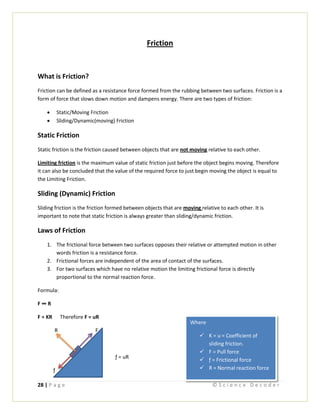Anzeige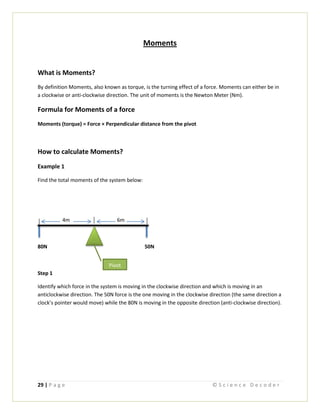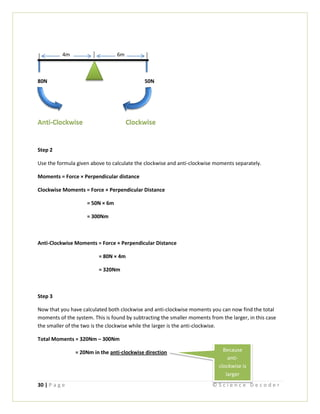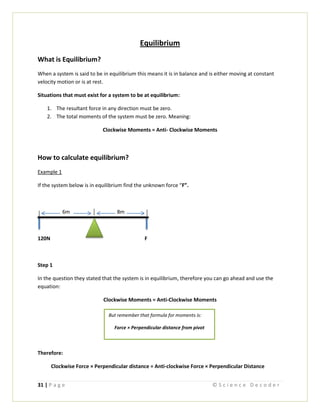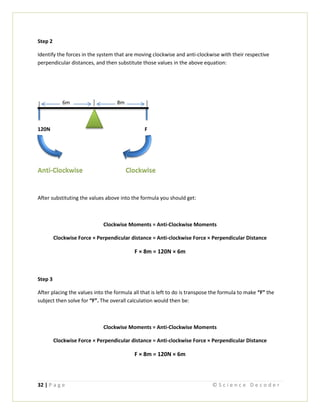Anzeige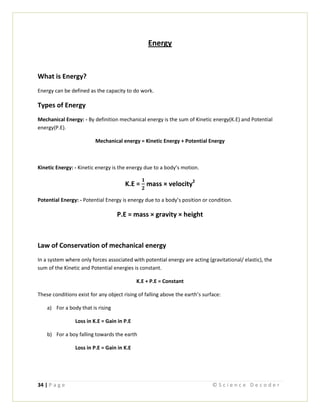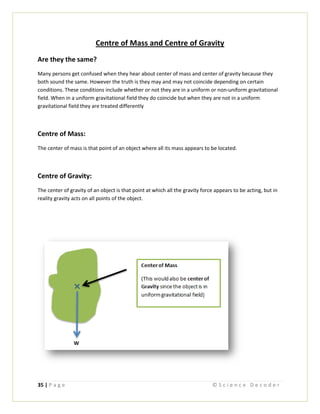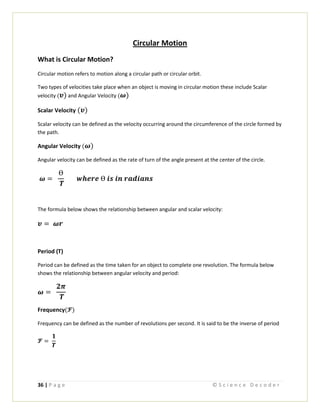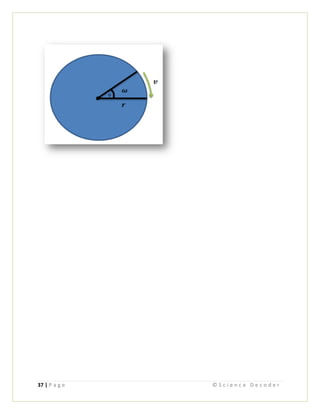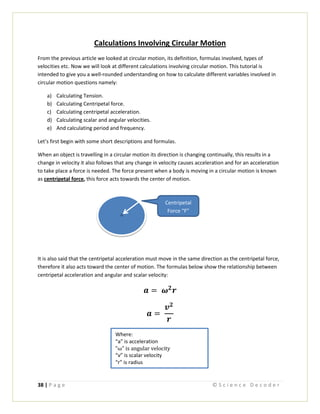Anzeige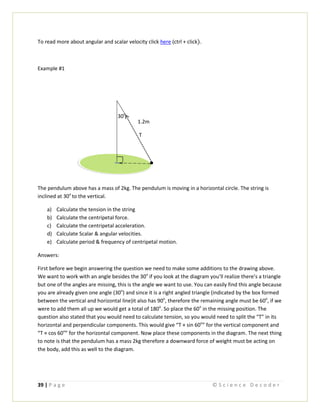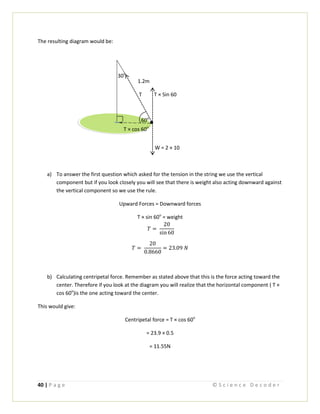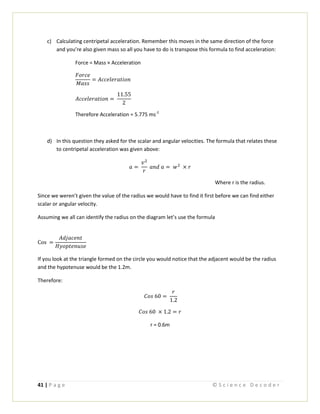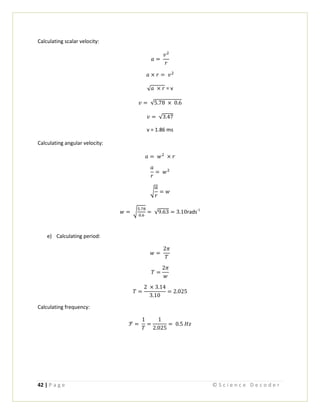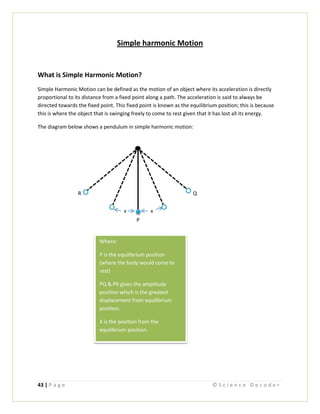Anzeige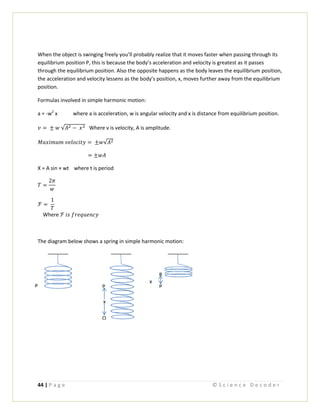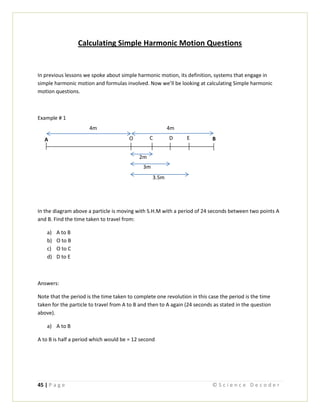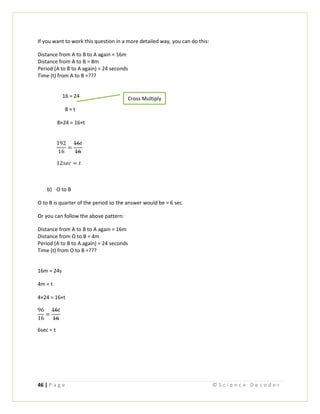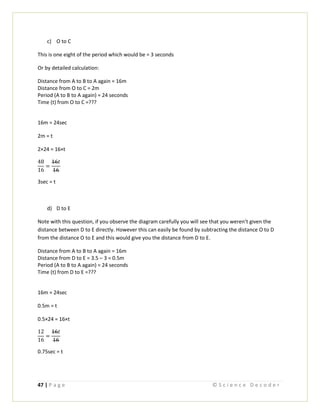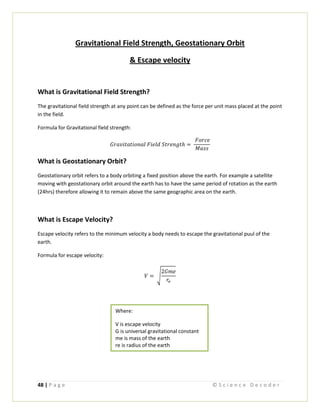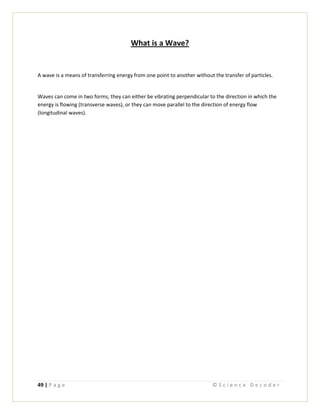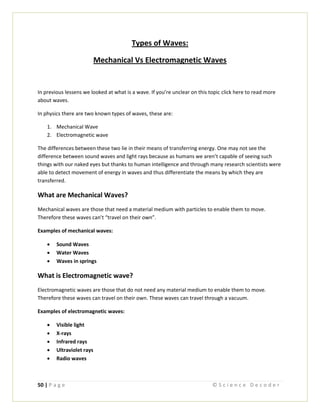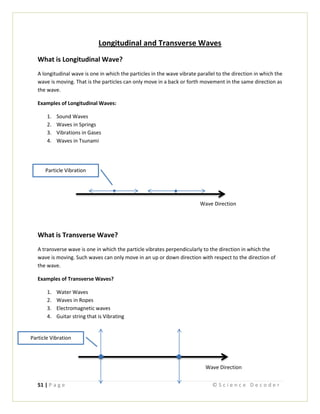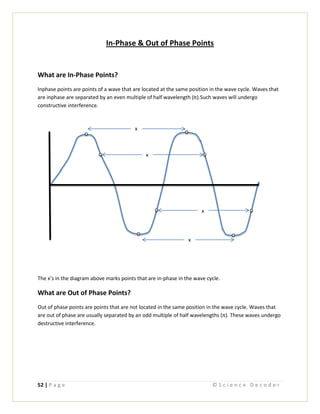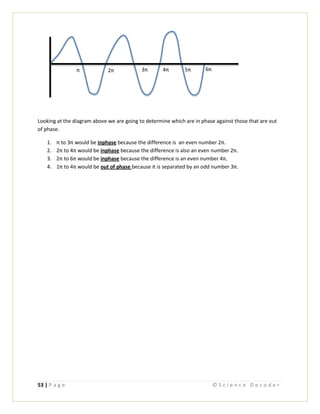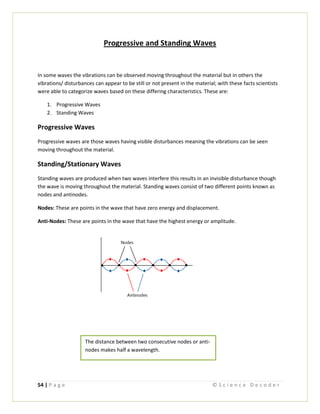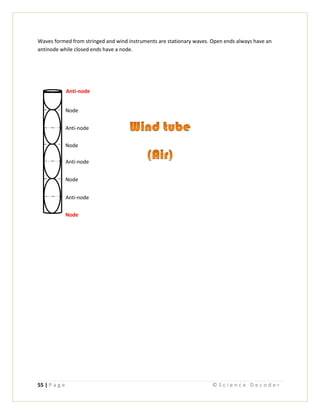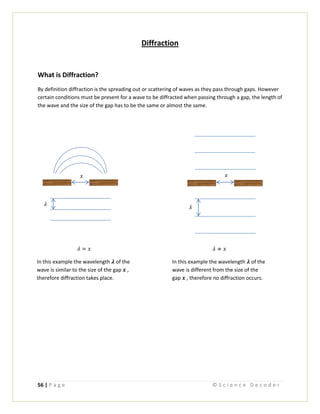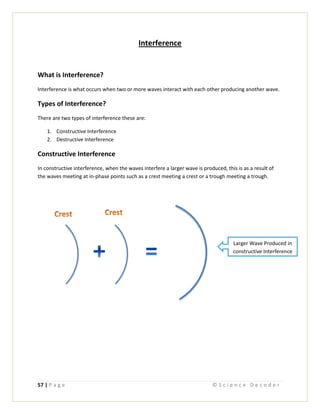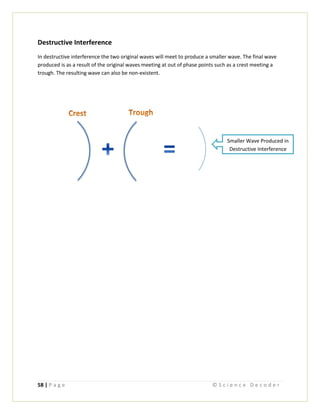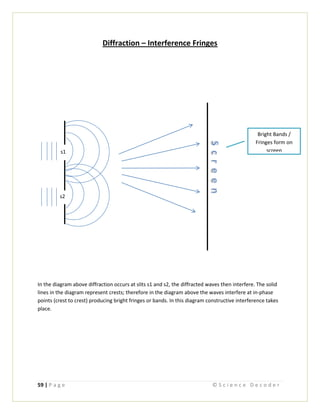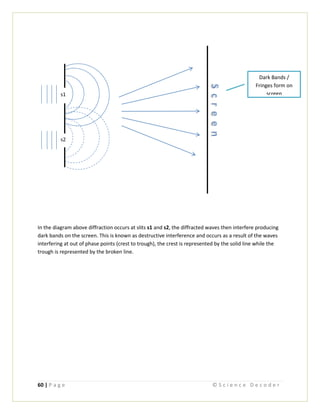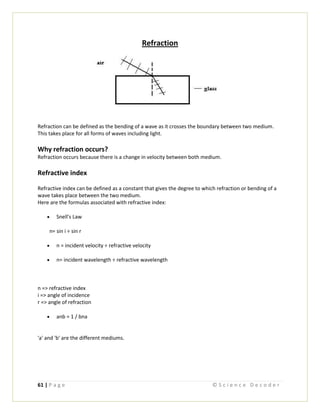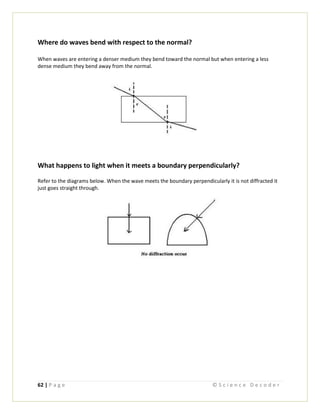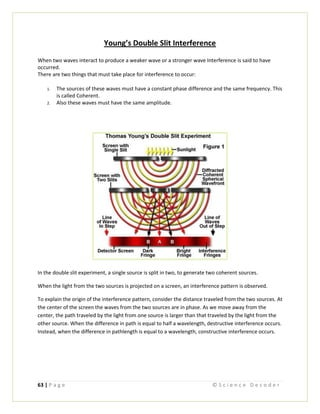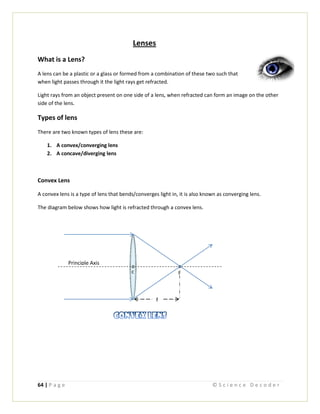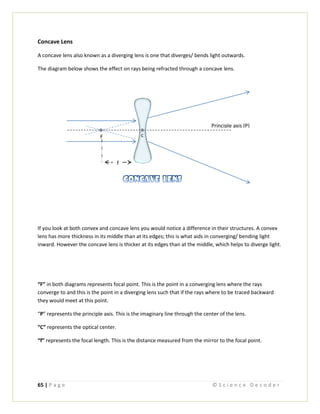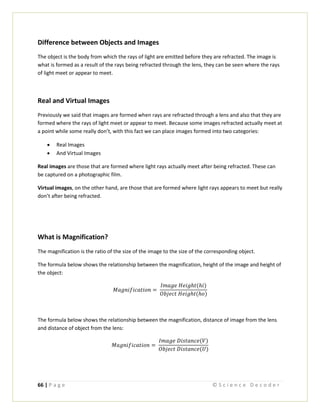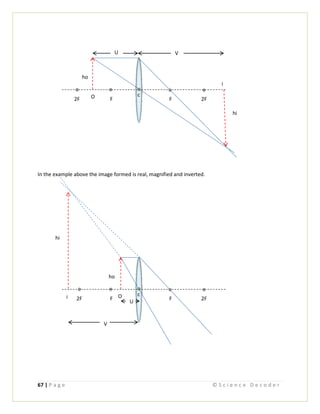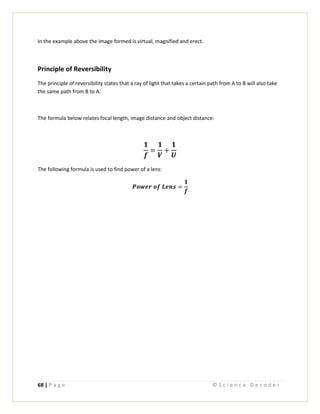Nächste SlideShare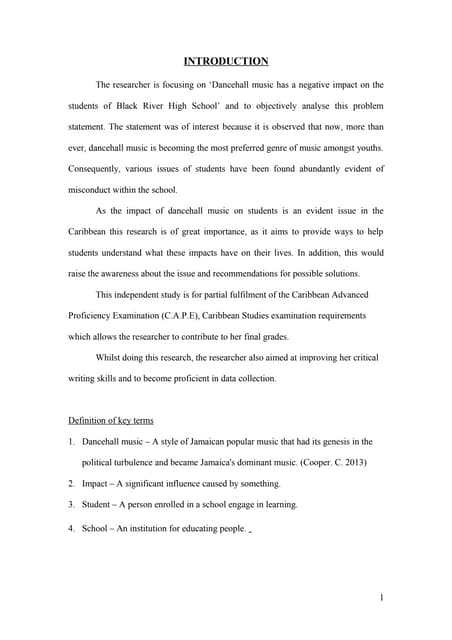Caribbean Studies Internal Assessment (Sample)
1 von 70
Anzeige

### Cape physics unit 1st edition

1. Cape Physics B y : S c i e n c e D e c o d e r A u t h o r : A . T h o m p s o n 8 / 2 7 / 2 0 1 1 Unit 1 Get more free notes at http://getyournotes.blogspot.com
2. 1 | P a g e © S c i e n c e D e c o d e r Content Topic Page About 2 Sources 3 Scalars and Vectors 4 Vector Addition and Subtraction 5 How to Draw vectors 6 Solving Vectors 7-8 Resolving Vectors 9-10  Questions & Answers 11-12 Newton’s Laws of Motion 13-14 Reaction Force Problems 15-19 Projectile Motion 20  Example 21-22 Momentum & Collisions 23-24 Impulse 25-27 Friction 28 Moments 29-30 Equilibrium 31-33 Energy 34 Centre of Mass & Gravity 35 Circular Motion 36-37  Examples 38-42 Simple Harmonic Motion 43-44  Examples 45-47 Geostationary Orbit… 48 Wave 49  Types of Waves 50  Longitudinal and Transverse 51  In-phase and Out-of-phase 52-53  Progressive and Standing 54-55  Diffraction 56  Interference 57-60  Refraction 61-62  Young’s Double Slit 63 Lenses 64-68
3. 2 | P a g e © S c i e n c e D e c o d e r About This e-book was created to give students a well-rounded understanding on difficult topics in advanced level physics. It was created based on the CAPE syllabus which is also compatible with other major syllabuses such as the A-Level syllabus. It was created mainly for students doing physics in their first year of college.
4. 3 | P a g e © S c i e n c e D e c o d e r Sources Most of the content present in this e-book was provided by Science Decoder, you can find us as at: http://getyournotes.blogspot.com The following articles were taken from the internet: Young’s Double Slit taken from: http://micro.magnet.fsu.edu/primer/java/scienceopticsu/interference/doubleslit/index.html http://vsg.quasihome.com/interfer.htm Recommended Sites: BiologyMad: - A very useful site containing a lot of biology notes that are covered in higher level biology such as A-Level and CAPE. Chemguide: - Another useful site which covers the hardest and less recognized topics in chemistry. In addition it has many other features and is highly recommended by other sites. A-Level Physics Notes: - As the name suggests it covers allot of topics in a-level.Allot of these topic are difficult but by just using this site as reference can greatly help you. They have allot of diagrams and explanations along with these notes
5. 4 | P a g e © S c i e n c e D e c o d e r Scalars and Vectors Scalars have magnitude only but Vectors have both magnitude and direction. The table below displays some of the most popular scalar and vector quantities. S.I Units Mass --> Grams (g), or Kilograms (Kg) Length --> Meter(m) Time --> Seconds(s)
6. 5 | P a g e © S c i e n c e D e c o d e r Addition and Subtraction Of Vectors The sum of two or more vectors is called a resultant.  When vectors are in the same directions we add. Example #1.  When vectors are in opposed directions we minus. Example #2
7. 6 | P a g e © S c i e n c e D e c o d e r How to Draw Vectors A Vector can be defined by capital letters at both ends or by one lower case letter.
8. 7 | P a g e © S c i e n c e D e c o d e r Solving Vectors Here is an example on how to solve a vector question: Example #1: A force of 3N acts at an angle of 90 degrees to a force of 4N. Find the magnitude and direction of their resultant R. (Note: a line similar to the 3N was drawn on right to make the vector tail to head. The resultant line R was then drawn from the tail of 4N line to Head of 3N line on right. Also note that direction in this case is the unknown angle.)
9. 8 | P a g e © S c i e n c e D e c o d e r Answer Using Pythagoras' theorem to find magnitude.
10. 9 | P a g e © S c i e n c e D e c o d e r Resolving Vectors Resolving vectors means splitting vectors into two perpendicular components. Resolving vectors can be very easy if you’re focused. Here I’ll be showing you how to resolve a vector and then solve its horizontal and vertical components. Using the vector below F it must first be split (resolved) into its vertical and horizontal components before it can be solved. Vector F Vector when resolved 1. Identify the component (line) adjacent to the given angle ‘A’ (here it is the horizontal component). 2. Label the adjacent component as F x COS A.
11. 10 | P a g e © S c i e n c e D e c o d e r 3. Identify the component opposite to the given angle ‘A’ (here it is the vertical component). 4. Label opposite component as F x SIN A. The following formulas can then be used to solve the components: Horizontal component = F x COS A Vertical component = F x SIN A Note: When resolving vectors the component adjacent to the given angle is ALWAYS the vector multiplied by the cosine (COS) of the angle while the component opposite the given angle is always the vector multiplied by the sin (SIN) of the angle.
12. 11 | P a g e © S c i e n c e D e c o d e r Resolving Vectors Questions and Answers In previous articles we covered the topic of resolving vectors, how to resolve a vector, definitions and a complete guide on how to go about splitting/resolving a vector into its perpendicular components and how to label them, in this article we will look at some examples on how to resolve vectors. Question # 1 Calculate the horizontal and vertical components of a 50N force which is acting 40 degrees to the horizontal. Answer: The question asked for the values of the horizontal and vertical components, so first you need to split the vector seen in the diagram above into its horizontal and vertical components: 40 0 40 0 Vertical Component Horizontal Component
13. 12 | P a g e © S c i e n c e D e c o d e r Now that you have identified both components the next thing that needs to be done is to label the components. Look at the diagram above and find out which of the components is adjacent to the given angle and label it “F×cosθ”, where F is the force and θ is the given angle. Also find out which component is opposite to the given angle and label it “F×sinθ”. In this example the component adjacent to the given angle is the horizontal component so it is labeled 50×cos 400 . The component opposite to the given angle is the vertical component so it is labeled 50×sin400 . Therefore your answer should be: Horizontal component = 50×cos400 = 38.30N Vertical Component = 50×sin400 = 32.14N 40 0 50×cos40 0 50×sin40 0
14. 13 | P a g e © S c i e n c e D e c o d e r Newton’s Laws of Motion Here are the three Newton’s Laws of motion: 1. Newton's first(1st) law of motion, 2. Second(2nd) Law of motion 3. And Newton's third (3rd) Law of motion. Newton’s first law of motion Newton’s 1st law of motion states that everybody continues in its state of rest or in constant velocity motion unless acted upon by an external force. Newton’s first law of motion simply means that no change in motion takes place unless an external force acts on the body. Newton’s second law of motion The rate of change of momentum of a body is directly proportional to the external force acting upon it. In its simplest form what newtons second law of motion is saying the acceleration on a body is directly proportional to the external force applied. The formulas relating to newtons second law of motion is: Force = mv - mu / t Or can be simplified as: F = m (v - u) / t but remember a = v - u / t Therefore F = ma Where F is force, m is mass, u and v are initial and final velocity respectively, t is time and a is acceleration.
15. 14 | P a g e © S c i e n c e D e c o d e r Newton’s third law motion Newtons 3rd law of motion states that if a body A exerts a force on a body B, then Body B will exert an equal but opposite force on Body A. Applications of Newton’s Laws of motion: 1st law: - When a car crashes the car stops but the person on the inside continues to move because there is no external force exerted on the person. 2nd Law: - Increase the force, by using gas, on the car and the acceleration will also increase. 3rd Law: - A bouncing ball applies a force to the ground and the ground applies an equal but opposite force on the ball.
16. 15 | P a g e © S c i e n c e D e c o d e r Laws of Motion & Reaction Force Problems In previous articles we discussednewton’s laws of motion and how they apply to life-like situations. In this article we’ll be using formulas/equations from the laws of motion and the laws themselves to solve various problems involving reaction force. By the end of this tutorial you should be able to: 1. Find the reaction force in a system 2. Apply newton’s laws of motion to various questions 3. Find missing variables such as acceleration when given values for other variables. Problem #1 A body of mass 7kg rest on a floor lift. Calculate the reaction force R exerted by the floor of the lift on the body if: a) The lift has an upward acceleration of 2m/s2 . b) The lift has a downward acceleration of 3m/s2 . c) The lift is moving with constant velocity. Answer: The first thing that needs to be done is to draw a diagram to represent the information in the question, doing this makes your calculations allot easier and reduces risk of errors. In questions like these where there’s a mass there is always a weight acting downward from the body; so the first thing to be drawn on the diagram is the weight (W) acting downward. Next is the reaction force(R), the reaction force always acts perpendicularly to the body. When done your diagram should look something like this: Floor Lift Body with mass 7kg
17. 16 | P a g e © S c i e n c e D e c o d e r a) This part of the question asked for the reaction force when the lift has an upward acceleration of 2m/s2 . When forces are moving in opposite directions you must subtract the smaller from the larger. In this question the reaction force is larger than the weight because the lift is moving in the upward direction. We’ll use the formula derived from newton’s laws of motion: Your answer should know look something like this: F = Mass × acceleration R – W = Mass × acceleration R – 70 = 7 x 2 R = 14 + 70 R = 84N Floor Lift Body with mass 7kg 2m/s2 Weight = mass x gravity Weight = 7 x 10 Weight = 70N
18. 17 | P a g e © S c i e n c e D e c o d e r b) This part of the question is very similar to the one previously done. The question asked for the reaction force when given a downward acceleration of 3m/s2 . In part “a” of the question the reaction force was larger because the lift was moving in an upward direction; therefore it also follows that the opposite should happen in this part of the question since the lift is now moving in the downward direction. Therefore the weight is larger than the reaction force. F = mass x acceleration 70 – R = Mass x acceleration 70 – R= 7 x 3 70 – R = 21 70 – 21 = R 49N = R c) In this part of the problem you’re required to find the reaction force given that the lift is moving at a constant velocity. Since it is moving at constant velocity acceleration would be zero and no motion is taking place. F = 0 R – W = 0 R – 70 = 0 R = 70N Floor Lift Body with mass 7kg 3m/s2 Note: Here the reaction force is equal to the weight
19. 18 | P a g e © S c i e n c e D e c o d e r Problem # 2 A body of mass 5kg is pulled up a smooth plane inclined at 300 to the horizontal by a force of 40N acting parallel to the plane. Calculate the acceleration of the body and the force exerted on it by the plane. Answers Before we begin calculating some other components must be added to the diagram. Remember in the previous question we said that where ever there’s a mass there is weight acting downwards. Also the weight must be resolved/split into its perpendicular components with respect to the plane. One of the components of weight is parallel to the plane while the other is perpendicular to the plane. Finally we must now add the reaction force. Remember that the reaction force must be perpendicular to the plane of the body. You may have noticed that another angle was added to the diagram, 600 , this was needed in order to find the perpendicular components of the weight. If you look at the diagram closely you’ll see that the line for the weight cuts the base of the triangle forming a 900 angle. Therefore the 60 degrees was found 300 ) 300 ) W = 5 x10 = 50N 60 0
20. 19 | P a g e © S c i e n c e D e c o d e r by subtracting the sum of the 90 degrees and 30 degrees from 180 giving 60 degrees. Because all angles in a triangle add up to 180. Now that we have labeled our diagram we can now begin answering the question. The first part asked for the acceleration of the body. Using newton’s second law F = Mass x Acceleration (a) 40 – W x cos60 = 5 x a 40 – 50 x cos60 = 5 x a 40 – 50(0.5) = 5 x a 40 – 25 = 5 x a 15 = 5 x a 15/5 = a 3m/s2 = a The next part of the question asked for the force exerted on the body by the plane, the force exerted is the reaction force. No motion is taking place in this direction therefore: Using Newton’s first law: F = 0 R – W x Sin60 = 0 R = W x Sin60 R = 50(0.8660) R = 43.30N Note: Remember that forces in opposite direction must be subtracted.
21. 20 | P a g e © S c i e n c e D e c o d e r Projectile Motion What is the projectile motion of a body? - A body in projectile motion will move in two dimensions simultaneously. Bodies that are in projectile motion are resolved (divided) into two components. A vertical component (Vy) and an horizontal component (Vx). These components however are dealt with simultaneously. Please refer to the diagram below showing projectile motion. Formulas associated with projectile motion include:  Vy = VsinA  Vx = VcosA  V = Sqrt [Vy^2 + Vx^2]  TanA = Vy / Vx => A = Tan^-1 A (Vy / Vx) Where A is used as angle instead of theta in diagram
22. 21 | P a g e © S c i e n c e D e c o d e r Example calculation involving projectile motion: 1. A body is projected with a velocity of 200m/s at an angle of 30 °C to the horizontal.  Calculate the time taken to reach its maximum height.  Calculate its velocity after 16 seconds. Answers:  Finding the time for maximum height. angle A = 30 °C V = 200m/s Acceleration(a) = -10 m/s2 Vy = 0 m/s Therefore Uy = VsinA Uy = 200 x Sin 30 Uy = 100 m/s Vy = Uy = at Vy - Uy = at a 0 - 100 = t - 10 10 sec = t Therefore Maximum Time (t) = 10 seconds  Finding the velocity after 16 seconds. Vx = V cos A Vx = 200 x Cos 30 Vx = 173.2 m/s Vy = Uy + at Vy = 100 - 10 (16) Note: Uy represents initial velocity
23. 22 | P a g e © S c i e n c e D e c o d e r Vy = -60 m/s Therefore V = √ [Vy^2 + Vx^2] = √ *(-60)^2 + (173.2)^2] = √33598 = 183.30 m/s
24. 23 | P a g e © S c i e n c e D e c o d e r Momentum and Types of Collision What is momentum? Momentum can be defined as the product of mass and velocity (m x v). Principle of conservation of momentum: The principle of conservation of momentum states that the total linear momentum of a system of interacting (colliding) bodies, on which no external force is acting remains constant. This principle is basically saying momentum before collision is equal to momentum after collision. Types of collisions: There are two types of collisions covered in physics they are Elastic and Inelastic collisions. Elastic collisions are those formed when the colliding bodies separate after collision, hence its name. When elastic collisions take place two things are conserved, Momentum and Kinetic Energy (K.E.), meaning none is lost from the system. Therefore in elastic collisions momentum before collision is equal to momentum after collision, and similarly Kinetic energy before collision is equal to kinetic energy after collision.
25. 24 | P a g e © S c i e n c e D e c o d e r In-elastic collisions refer to collisions in which the objects remain or stick together after collision. Seeing that the objects remain together after collision Kinetic energy is therefore not conserved only momentum is conserved.
26. 25 | P a g e © S c i e n c e D e c o d e r Impulse What is Impulse? Impulse can be defined as the force per unit time or change in momentum. Momentum is changed whenever a force is applied to a body. From these definitions one can already see what formulas are in relation to impulse. Formulas associated with Impulse: Impulse = Force x Time Or Impulse = Change in momentum= mV – mU Where ‘m’ is Mass and V, U is final and initial velocity respectively. Below are some calculations involving impulse. Here you’ll be using the formulas above to find the missing variable. Example 1. A force of 100 N is applied for 8 seconds. What is the impulse? Answer: Right away you can easily solve for impulse using the first formula above because all other variables are given. Therefore Impulse = Force x Time = 100N x 8sec = 800Ns Example 2. An impulse of 250Ns is applied for 10 seconds. What is the applied force. Answer: Again you can see that you have to use the first formula above, but in this case they gave values for impulse and time, therefore all you have to do is transpose the formula to make Force the subject and then solve for Force.
27. 26 | P a g e © S c i e n c e D e c o d e r Therefore Impulse = Force x Time Force = 25 N Example 3. A body of 4kg is moving at 5m/s when it is given an impulse of 8Ns in the direction of the motion. a) What is the Velocity of the body immediately after the impulse? b) If the impulse acts for 0.02 seconds. What is the average value of the force exerted? Answers: a) Here they gave you mass (4kg), initial velocity (5m/s) and impulse (8Ns). Therefore all you need to do is transpose the second formula, which relates impulse to momentum, to make the final velocity(V) the subject of the formula and then solve. Therefore Impulse = mV - mU Impulse + mU = mV – mU + mU Impulse + mU = mV
28. 27 | P a g e © S c i e n c e D e c o d e r b) All you need to do is transpose for force and then solve. When done transposing you should get: Therefore Force = 400N
29. 28 | P a g e © S c i e n c e D e c o d e r Friction What is Friction? Friction can be defined as a resistance force formed from the rubbing between two surfaces. Friction is a form of force that slows down motion and dampens energy. There are two types of friction:  Static/Moving Friction  Sliding/Dynamic(moving) Friction Static Friction Static friction is the friction caused between objects that are not moving relative to each other. Limiting friction is the maximum value of static friction just before the object begins moving. Therefore it can also be concluded that the value of the required force to just begin moving the object is equal to the Limiting Friction. Sliding (Dynamic) Friction Sliding friction is the friction formed between objects that are moving relative to each other. It is important to note that static friction is always greater than sliding/dynamic friction. Laws of Friction 1. The frictional force between two surfaces opposes their relative or attempted motion in other words friction is a resistance force. 2. Frictional forces are independent of the area of contact of the surfaces. 3. For two surfaces which have no relative motion the limiting frictional force is directly proportional to the normal reaction force. Formula: F ∞ R F = KR Therefore F = uR R F ƒ = uR ƒ Where  K = u = Coefficient of sliding friction.  F = Pull force  ƒ = Frictional force  R = Normal reaction force
30. 29 | P a g e © S c i e n c e D e c o d e r Moments What is Moments? By definition Moments, also known as torque, is the turning effect of a force. Moments can either be in a clockwise or anti-clockwise direction. The unit of moments is the Newton Meter (Nm). Formula for Moments of a force Moments (torque) = Force × Perpendicular distance from the pivot How to calculate Moments? Example 1 Find the total moments of the system below: 4m 6m 80N 50N Step 1 Identify which force in the system is moving in the clockwise direction and which is moving in an anticlockwise direction. The 50N force is the one moving in the clockwise direction (the same direction a clock’s pointer would move) while the 80N is moving in the opposite direction (anti-clockwise direction). Pivot
31. 30 | P a g e © S c i e n c e D e c o d e r 4m 6m 80N 50N Anti-Clockwise Clockwise Step 2 Use the formula given above to calculate the clockwise and anti-clockwise moments separately. Moments = Force × Perpendicular distance Clockwise Moments = Force × Perpendicular Distance = 50N × 6m = 300Nm Anti-Clockwise Moments = Force × Perpendicular Distance = 80N × 4m = 320Nm Step 3 Now that you have calculated both clockwise and anti-clockwise moments you can now find the total moments of the system. This is found by subtracting the smaller moments from the larger, in this case the smaller of the two is the clockwise while the larger is the anti-clockwise. Total Moments = 320Nm – 300Nm = 20Nm in the anti-clockwise direction Because anti- clockwise is larger
32. 31 | P a g e © S c i e n c e D e c o d e r Equilibrium What is Equilibrium? When a system is said to be in equilibrium this means it is in balance and is either moving at constant velocity motion or is at rest. Situations that must exist for a system to be at equilibrium: 1. The resultant force in any direction must be zero. 2. The total moments of the system must be zero. Meaning: Clockwise Moments = Anti- Clockwise Moments How to calculate equilibrium? Example 1 If the system below is in equilibrium find the unknown force “F”. 6m 8m 120N F Step 1 In the question they stated that the system is in equilibrium, therefore you can go ahead and use the equation: Clockwise Moments = Anti-Clockwise Moments Therefore: Clockwise Force × Perpendicular distance = Anti-clockwise Force × Perpendicular Distance But remember that formula for moments is: Force × Perpendicular distance from pivot
33. 32 | P a g e © S c i e n c e D e c o d e r Step 2 Identify the forces in the system that are moving clockwise and anti-clockwise with their respective perpendicular distances, and then substitute those values in the above equation: 6m 8m 120N F Anti-Clockwise Clockwise After substituting the values above into the formula you should get: Clockwise Moments = Anti-Clockwise Moments Clockwise Force × Perpendicular distance = Anti-clockwise Force × Perpendicular Distance F × 8m = 120N × 6m Step 3 After placing the values into the formula all that is left to do is transpose the formula to make “F” the subject then solve for “F”. The overall calculation would then be: Clockwise Moments = Anti-Clockwise Moments Clockwise Force × Perpendicular distance = Anti-clockwise Force × Perpendicular Distance F × 8m = 120N × 6m
34. 33 | P a g e © S c i e n c e D e c o d e r F = 90N
35. 34 | P a g e © S c i e n c e D e c o d e r Energy What is Energy? Energy can be defined as the capacity to do work. Types of Energy Mechanical Energy: - By definition mechanical energy is the sum of Kinetic energy(K.E) and Potential energy(P.E). Mechanical energy = Kinetic Energy + Potential Energy Kinetic Energy: - Kinetic energy is the energy due to a body’s motion. K.E = mass × velocity2 Potential Energy: - Potential Energy is energy due to a body’s position or condition. P.E = mass × gravity × height Law of Conservation of mechanical energy In a system where only forces associated with potential energy are acting (gravitational/ elastic), the sum of the Kinetic and Potential energies is constant. K.E + P.E = Constant These conditions exist for any object rising of falling above the earth’s surface: a) For a body that is rising Loss in K.E = Gain in P.E b) For a boy falling towards the earth Loss in P.E = Gain in K.E
36. 35 | P a g e © S c i e n c e D e c o d e r Centre of Mass and Centre of Gravity Are they the same? Many persons get confused when they hear about center of mass and center of gravity because they both sound the same. However the truth is they may and may not coincide depending on certain conditions. These conditions include whether or not they are in a uniform or non-uniform gravitational field. When in a uniform gravitational field they do coincide but when they are not in a uniform gravitational field they are treated differently Centre of Mass: The center of mass is that point of an object where all its mass appears to be located. Centre of Gravity: The center of gravity of an object is that point at which all the gravity force appears to be acting, but in reality gravity acts on all points of the object.
37. 36 | P a g e © S c i e n c e D e c o d e r Circular Motion What is Circular Motion? Circular motion refers to motion along a circular path or circular orbit. Two types of velocities take place when an object is moving in circular motion these include Scalar velocity and Angular Velocity Scalar Velocity Scalar velocity can be defined as the velocity occurring around the circumference of the circle formed by the path. Angular Velocity Angular velocity can be defined as the rate of turn of the angle present at the center of the circle. The formula below shows the relationship between angular and scalar velocity: Period (T) Period can be defined as the time taken for an object to complete one revolution. The formula below shows the relationship between angular velocity and period: Frequency Frequency can be defined as the number of revolutions per second. It is said to be the inverse of period
38. 37 | P a g e © S c i e n c e D e c o d e r
39. 38 | P a g e © S c i e n c e D e c o d e r Calculations Involving Circular Motion From the previous article we looked at circular motion, its definition, formulas involved, types of velocities etc. Now we will look at different calculations involving circular motion. This tutorial is intended to give you a well-rounded understanding on how to calculate different variables involved in circular motion questions namely: a) Calculating Tension. b) Calculating Centripetal force. c) Calculating centripetal acceleration. d) Calculating scalar and angular velocities. e) And calculating period and frequency. Let’s first begin with some short descriptions and formulas. When an object is travelling in a circular motion its direction is changing continually, this results in a change in velocity it also follows that any change in velocity causes acceleration and for an acceleration to take place a force is needed. The force present when a body is moving in a circular motion is known as centripetal force, this force acts towards the center of motion. It is also said that the centripetal acceleration must move in the same direction as the centripetal force, therefore it also acts toward the center of motion. The formulas below show the relationship between centripetal acceleration and angular and scalar velocity: Centripetal Force “F” "ω" is angular velocity Where: “a” is acceleration “v” is scalar velocity “r” is radius
41. 40 | P a g e © S c i e n c e D e c o d e r The resulting diagram would be: 1.2m T T × Sin 60 W = 2 × 10 a) To answer the first question which asked for the tension in the string we use the vertical component but if you look closely you will see that there is weight also acting downward against the vertical component so we use the rule. Upward Forces = Downward forces T × sin 60o = weight sin b) Calculating centripetal force. Remember as stated above that this is the force acting toward the center. Therefore if you look at the diagram you will realize that the horizontal component ( T × cos 60o )is the one acting toward the center. This would give: Centripetal force = T × cos 60o = 23.9 × 0.5 = 11.55N 30o 60o T × cos 60o
42. 41 | P a g e © S c i e n c e D e c o d e r c) Calculating centripetal acceleration. Remember this moves in the same direction of the force and you’re also given mass so all you have to do is transpose this formula to find acceleration: Force = Mass × Acceleration Therefore Acceleration = 5.775 ms-2 d) In this question they asked for the scalar and angular velocities. The formula that relates these to centripetal acceleration was given above: Where r is the radius. Since we weren’t given the value of the radius we would have to find it first before we can find either scalar or angular velocity. Assuming we all can identify the radius on the diagram let’s use the formula os If you look at the triangle formed on the circle you would notice that the adjacent would be the radius and the hypotenuse would be the 1.2m. Therefore: r = 0.6m
43. 42 | P a g e © S c i e n c e D e c o d e r Calculating scalar velocity: √ = v √ √ v = 1.86 ms Calculating angular velocity: √ √ √ rads-1 e) Calculating period: Calculating frequency:
44. 43 | P a g e © S c i e n c e D e c o d e r Simple harmonic Motion What is Simple Harmonic Motion? Simple Harmonic Motion can be defined as the motion of an object where its acceleration is directly proportional to its distance from a fixed point along a path. The acceleration is said to always be directed towards the fixed point. This fixed point is known as the equilibrium position; this is because this is where the object that is swinging freely to come to rest given that it has lost all its energy. The diagram below shows a pendulum in simple harmonic motion: R Q P x x Where: P is the equilibrium position (where the body would come to rest) PQ & PR gives the amplitude position which is the greatest displacement from equilibrium position. X is the position from the equilibrium position.
45. 44 | P a g e © S c i e n c e D e c o d e r When the object is swinging freely you’ll probably realize that it moves faster when passing through its equilibrium position P, this is because the body’s acceleration and velocity is greatest as it passes through the equilibrium position. Also the opposite happens as the body leaves the equilibrium position, the acceleration and velocity lessens as the body’s position, x, moves further away from the equilibrium position. Formulas involved in simple harmonic motion: a = -w2 x where a is acceleration, w is angular velocity and x is distance from equilibrium position. √ Where v is velocity, A is amplitude. √ X = A sin × wt where t is period Where The diagram below shows a spring in simple harmonic motion: P P Q x P R x
46. 45 | P a g e © S c i e n c e D e c o d e r Calculating Simple Harmonic Motion Questions In previous lessons we spoke about simple harmonic motion, its definition, systems that engage in simple harmonic motion and formulas involved. Now we’ll be looking at calculating Simple harmonic motion questions. Example # 1 In the diagram above a particle is moving with S.H.M with a period of 24 seconds between two points A and B. Find the time taken to travel from: a) A to B b) O to B c) O to C d) D to E Answers: Note that the period is the time taken to complete one revolution in this case the period is the time taken for the particle to travel from A to B and then to A again (24 seconds as stated in the question above). a) A to B A to B is half a period which would be = 12 second A O C D E B 4m 4m 2m 3m 3.5m
47. 46 | P a g e © S c i e n c e D e c o d e r If you want to work this question in a more detailed way, you can do this: Distance from A to B to A again = 16m Distance from A to B = 8m Period (A to B to A again) = 24 seconds Time (t) from A to B =??? 16 = 24 8 = t 8×24 = 16×t b) O to B O to B is quarter of the period so the answer would be = 6 sec Or you can follow the above pattern: Distance from A to B to A again = 16m Distance from O to B = 4m Period (A to B to A again) = 24 seconds Time (t) from O to B =??? 16m = 24s 4m = t 4×24 = 16×t 6sec = t Cross Multiply
48. 47 | P a g e © S c i e n c e D e c o d e r c) O to C This is one eight of the period which would be = 3 seconds Or by detailed calculation: Distance from A to B to A again = 16m Distance from O to C = 2m Period (A to B to A again) = 24 seconds Time (t) from O to C =??? 16m = 24sec 2m = t 2×24 = 16×t 3sec = t d) D to E Note with this question, if you observe the diagram carefully you will see that you weren’t given the distance between D to E directly. However this can easily be found by subtracting the distance O to D from the distance O to E and this would give you the distance from D to E. Distance from A to B to A again = 16m Distance from D to E = 3.5 – 3 = 0.5m Period (A to B to A again) = 24 seconds Time (t) from D to E =??? 16m = 24sec 0.5m = t 0.5×24 = 16×t 0.75sec = t
49. 48 | P a g e © S c i e n c e D e c o d e r Gravitational Field Strength, Geostationary Orbit & Escape velocity What is Gravitational Field Strength? The gravitational field strength at any point can be defined as the force per unit mass placed at the point in the field. Formula for Gravitational field strength: What is Geostationary Orbit? Geostationary orbit refers to a body orbiting a fixed position above the earth. For example a satellite moving with geostationary orbit around the earth has to have the same period of rotation as the earth (24hrs) therefore allowing it to remain above the same geographic area on the earth. What is Escape Velocity? Escape velocity refers to the minimum velocity a body needs to escape the gravitational puul of the earth. Formula for escape velocity: √ Where: V is escape velocity G is universal gravitational constant me is mass of the earth re is radius of the earth
50. 49 | P a g e © S c i e n c e D e c o d e r What is a Wave? A wave is a means of transferring energy from one point to another without the transfer of particles. Waves can come in two forms, they can either be vibrating perpendicular to the direction in which the energy is flowing (transverse waves), or they can move parallel to the direction of energy flow (longitudinal waves).
51. 50 | P a g e © S c i e n c e D e c o d e r Types of Waves: Mechanical Vs Electromagnetic Waves In previous lessens we looked at what is a wave. If you’re unclear on this topic click here to read more about waves. In physics there are two known types of waves, these are: 1. Mechanical Wave 2. Electromagnetic wave The differences between these two lie in their means of transferring energy. One may not see the difference between sound waves and light rays because as humans we aren’t capable of seeing such things with our naked eyes but thanks to human intelligence and through many research scientists were able to detect movement of energy in waves and thus differentiate the means by which they are transferred. What are Mechanical Waves? Mechanical waves are those that need a material medium with particles to enable them to move. Therefore these waves can’t “travel on their own”. Examples of mechanical waves:  Sound Waves  Water Waves  Waves in springs What is Electromagnetic wave? Electromagnetic waves are those that do not need any material medium to enable them to move. Therefore these waves can travel on their own. These waves can travel through a vacuum. Examples of electromagnetic waves:  Visible light  X-rays  Infrared rays  Ultraviolet rays  Radio waves
52. 51 | P a g e © S c i e n c e D e c o d e r Longitudinal and Transverse Waves What is Longitudinal Wave? A longitudinal wave is one in which the particles in the wave vibrate parallel to the direction in which the wave is moving. That is the particles can only move in a back or forth movement in the same direction as the wave. Examples of Longitudinal Waves: 1. Sound Waves 2. Waves in Springs 3. Vibrations in Gases 4. Waves in Tsunami What is Transverse Wave? A transverse wave is one in which the particle vibrates perpendicularly to the direction in which the wave is moving. Such waves can only move in an up or down direction with respect to the direction of the wave. Examples of Transverse Waves? 1. Water Waves 2. Waves in Ropes 3. Electromagnetic waves 4. Guitar string that is Vibrating Wave Direction Particle Vibration Wave Direction Particle Vibration
53. 52 | P a g e © S c i e n c e D e c o d e r In-Phase & Out of Phase Points What are In-Phase Points? Inphase points are points of a wave that are located at the same position in the wave cycle. Waves that are inphase are separated by an even multiple of half wavelength (π).Such waves will undergo constructive interference. The x’s in the diagram above marks points that are in-phase in the wave cycle. What are Out of Phase Points? Out of phase points are points that are not located in the same position in the wave cycle. Waves that are out of phase are usually separated by an odd multiple of half wavelengths (π). These waves undergo destructive interference. x x x x
54. 53 | P a g e © S c i e n c e D e c o d e r Looking at the diagram above we are going to determine which are in phase against those that are out of phase. 1. π to 3π would be inphase because the difference is an even number 2π. 2. 2π to 4π would be inphase because the difference is also an even number 2π. 3. 2π to 6π would be inphase because the difference is an even number 4π. 4. 1π to 4π would be out of phase because it is separated by an odd number 3π. π 2π 3π 4π 5π 6π
55. 54 | P a g e © S c i e n c e D e c o d e r Progressive and Standing Waves In some waves the vibrations can be observed moving throughout the material but in others the vibrations/ disturbances can appear to be still or not present in the material; with these facts scientists were able to categorize waves based on these differing characteristics. These are: 1. Progressive Waves 2. Standing Waves Progressive Waves Progressive waves are those waves having visible disturbances meaning the vibrations can be seen moving throughout the material. Standing/Stationary Waves Standing waves are produced when two waves interfere this results in an invisible disturbance though the wave is moving throughout the material. Standing waves consist of two different points known as nodes and antinodes. Nodes: These are points in the wave that have zero energy and displacement. Anti-Nodes: These are points in the wave that have the highest energy or amplitude. The distance between two consecutive nodes or anti- nodes makes half a wavelength.
56. 55 | P a g e © S c i e n c e D e c o d e r Waves formed from stringed and wind instruments are stationary waves. Open ends always have an antinode while closed ends have a node. Node Node Node Node Anti-node Anti-node Anti-node Anti-node
57. 56 | P a g e © S c i e n c e D e c o d e r Diffraction What is Diffraction? By definition diffraction is the spreading out or scattering of waves as they pass through gaps. However certain conditions must be present for a wave to be diffracted when passing through a gap, the length of the wave and the size of the gap has to be the same or almost the same. 𝜆 ≃ 𝑥 In this example the wavelength 𝝀 of the wave is similar to the size of the gap 𝒙 , therefore diffraction takes place. 𝜆 ≄ 𝑥 In this example the wavelength 𝝀 of the wave is different from the size of the gap 𝒙 , therefore no diffraction occurs. 𝑥 𝑥 𝜆 𝜆
58. 57 | P a g e © S c i e n c e D e c o d e r Interference What is Interference? Interference is what occurs when two or more waves interact with each other producing another wave. Types of Interference? There are two types of interference these are: 1. Constructive Interference 2. Destructive Interference Constructive Interference In constructive interference, when the waves interfere a larger wave is produced, this is as a result of the waves meeting at in-phase points such as a crest meeting a crest or a trough meeting a trough. Larger Wave Produced in constructive Interference
59. 58 | P a g e © S c i e n c e D e c o d e r Destructive Interference In destructive interference the two original waves will meet to produce a smaller wave. The final wave produced is as a result of the original waves meeting at out of phase points such as a crest meeting a trough. The resulting wave can also be non-existent. Smaller Wave Produced in Destructive Interference
60. 59 | P a g e © S c i e n c e D e c o d e r Diffraction – Interference Fringes In the diagram above diffraction occurs at slits s1 and s2, the diffracted waves then interfere. The solid lines in the diagram represent crests; therefore in the diagram above the waves interfere at in-phase points (crest to crest) producing bright fringes or bands. In this diagram constructive interference takes place. Bright Bands / Fringes form on screens1 s2
61. 60 | P a g e © S c i e n c e D e c o d e r In the diagram above diffraction occurs at slits s1 and s2, the diffracted waves then interfere producing dark bands on the screen. This is known as destructive interference and occurs as a result of the waves interfering at out of phase points (crest to trough), the crest is represented by the solid line while the trough is represented by the broken line. Dark Bands / Fringes form on screens1 s2
62. 61 | P a g e © S c i e n c e D e c o d e r Refraction Refraction can be defined as the bending of a wave as it crosses the boundary between two medium. This takes place for all forms of waves including light. Why refraction occurs? Refraction occurs because there is a change in velocity between both medium. Refractive index Refractive index can be defined as a constant that gives the degree to which refraction or bending of a wave takes place between the two medium. Here are the formulas associated with refractive index:  Snell's Law n= sin i ÷ sin r  n = incident velocity ÷ refractive velocity  n= incident wavelength ÷ refractive wavelength n => refractive index i => angle of incidence r => angle of refraction  anb = 1 / bna 'a' and 'b' are the different mediums.
63. 62 | P a g e © S c i e n c e D e c o d e r Where do waves bend with respect to the normal? When waves are entering a denser medium they bend toward the normal but when entering a less dense medium they bend away from the normal. What happens to light when it meets a boundary perpendicularly? Refer to the diagrams below. When the wave meets the boundary perpendicularly it is not diffracted it just goes straight through.
64. 63 | P a g e © S c i e n c e D e c o d e r Young’s Double Slit Interference When two waves interact to produce a weaker wave or a stronger wave Interference is said to have occurred. There are two things that must take place for interference to occur: 1. The sources of these waves must have a constant phase difference and the same frequency. This is called Coherent. 2. Also these waves must have the same amplitude. In the double slit experiment, a single source is split in two, to generate two coherent sources. When the light from the two sources is projected on a screen, an interference pattern is observed. To explain the origin of the interference pattern, consider the distance traveled from the two sources. At the center of the screen the waves from the two sources are in phase. As we move away from the center, the path traveled by the light from one source is larger than that traveled by the light from the other source. When the difference in path is equal to half a wavelength, destructive interference occurs. Instead, when the difference in pathlength is equal to a wavelength, constructive interference occurs.
65. 64 | P a g e © S c i e n c e D e c o d e r Lenses What is a Lens? A lens can be a plastic or a glass or formed from a combination of these two such that when light passes through it the light rays get refracted. Light rays from an object present on one side of a lens, when refracted can form an image on the other side of the lens. Types of lens There are two known types of lens these are: 1. A convex/converging lens 2. A concave/diverging lens Convex Lens A convex lens is a type of lens that bends/converges light in, it is also known as converging lens. The diagram below shows how light is refracted through a convex lens. c F Principle Axis f
66. 65 | P a g e © S c i e n c e D e c o d e r Concave Lens A concave lens also known as a diverging lens is one that diverges/ bends light outwards. The diagram below shows the effect on rays being refracted through a concave lens. If you look at both convex and concave lens you would notice a difference in their structures. A convex lens has more thickness in its middle than at its edges; this is what aids in converging/ bending light inward. However the concave lens is thicker at its edges than at the middle, which helps to diverge light. “F” in both diagrams represents focal point. This is the point in a converging lens where the rays converge to and this is the point in a diverging lens such that if the rays where to be traced backward they would meet at this point. “P” represents the principle axis. This is the imaginary line through the center of the lens. “C” represents the optical center. “f” represents the focal length. This is the distance measured from the mirror to the focal point. cF f Principle axis (P)
67. 66 | P a g e © S c i e n c e D e c o d e r Difference between Objects and Images The object is the body from which the rays of light are emitted before they are refracted. The image is what is formed as a result of the rays being refracted through the lens, they can be seen where the rays of light meet or appear to meet. Real and Virtual Images Previously we said that images are formed when rays are refracted through a lens and also that they are formed where the rays of light meet or appear to meet. Because some images refracted actually meet at a point while some really don’t, with this fact we can place images formed into two categories:  Real Images  And Virtual Images Real images are those that are formed where light rays actually meet after being refracted. These can be captured on a photographic film. Virtual images, on the other hand, are those that are formed where light rays appears to meet but really don’t after being refracted. What is Magnification? The magnification is the ratio of the size of the image to the size of the corresponding object. The formula below shows the relationship between the magnification, height of the image and height of the object: The formula below shows the relationship between the magnification, distance of image from the lens and distance of object from the lens:
68. 67 | P a g e © S c i e n c e D e c o d e r In the example above the image formed is real, magnified and inverted. c F F2F 2FO ho hi i U V c F F2F 2FO ho hi i U V
69. 68 | P a g e © S c i e n c e D e c o d e r In the example above the image formed is virtual, magnified and erect. Principle of Reversibility The principle of reversibility states that a ray of light that takes a certain path from A to B will also take the same path from B to A. The formula below relates focal length, image distance and object distance: The following formula is used to find power of a lens:
70. 69 | P a g e © S c i e n c e D e c o d e r
Anzeige